Representations of 3D: Plot3D

This is a function to give the magnitude of the electrostatic potential at a point x,y,z for a point charge placed at xo,yo.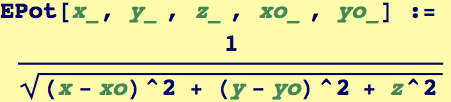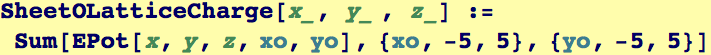SheetOLatticeCharge represents the electric field produced by an 11 by 11 array of point charges arranged on the x-y plane at z = 0. The following command evaluates and plots the field variation in the plane z = 0.25: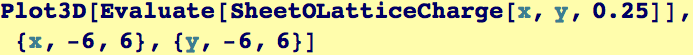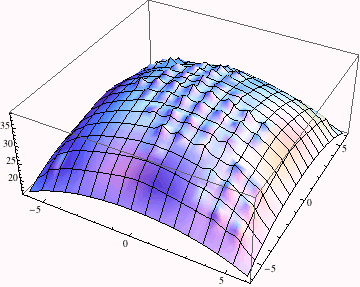Note below how theplot is set to contain the output of the Plot3D command---it is now a symbol assigned to a graphics object.  The number of plotpoints is increased so that we can resolve all the bumps.  This will take a while to compute on most machines.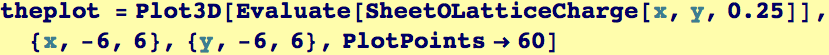This demonstrates the use of RegionFunction plot option which is pure function. Here, only the region inside a cylinder with radius 9 (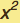+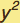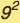) is plotted.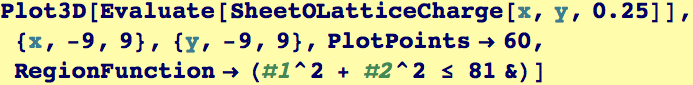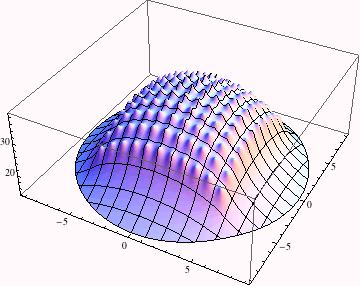This demonstrates the use of the ColorFunction  plot option which is pure function. Here we use one of Mathematica ColorData functions.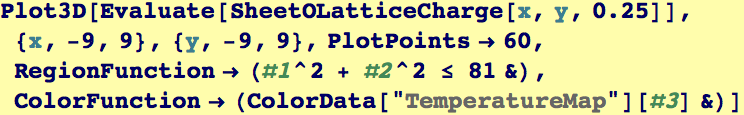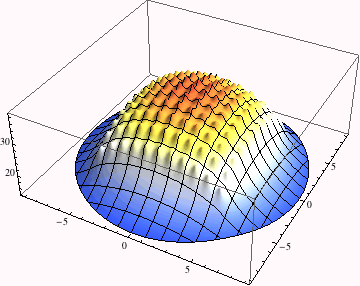Created by Wolfram Mathematica 6.0  (28 August 2007)